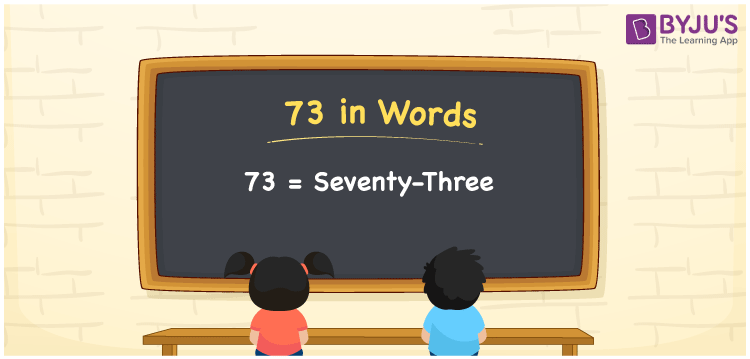# 73 in Words

The number 73 in words is expressed as seventy-three. The number 73 is a cardinal number, as it is used to express the value or it helps in the counting of things. For example, the cost of the book is Rs. 73. The place value system is employed to convert the number into words. Here is a complete explanation of writing the number 73 in words.

 73 in Words: Seventy-three. Seventy-three in Numerical Form: 73.

## 73 in English Words## How to Write 73 in Words?

As discussed above, the place value chart is helpful for writing the number 73 in words. The number 73 is a two-digit number, and it is expressed as follows.

 Tens Ones 7 3

The expanded form of 73 is as follows:

= 7 × Ten + 3 × One

= 7 × 10 + 3 × 1

= 70 + 3

= 73

= seventy-three

Hence, 73 in words is seventy-three.

73 is a natural number that lies between 72 and 74.

73 in words – Seventy-three

Is 73 an odd number? – Yes

Is 73 an even number? – No

Is 73 a perfect square number? – No

Is 73 a perfect cube number? – No

Is 73 a prime number? – Yes

Is 73 a composite number? – No

## Frequently Asked Questions on 73 in Words

Q1

### How to write 73 in words?

73 in words is seventy-three.

Q2

### Simplify 70 + 3, and express it in words.

Simplifying 70 + 3, we get 73. Hence, 73 in words is seventy-three.

Q3

### Is 73 a prime number?

Yes, 73 is a prime number.